# DIY Basketball Scoreboard

A professional-level scoreboard featuring a wireless controller, bright lights, and a loud horn.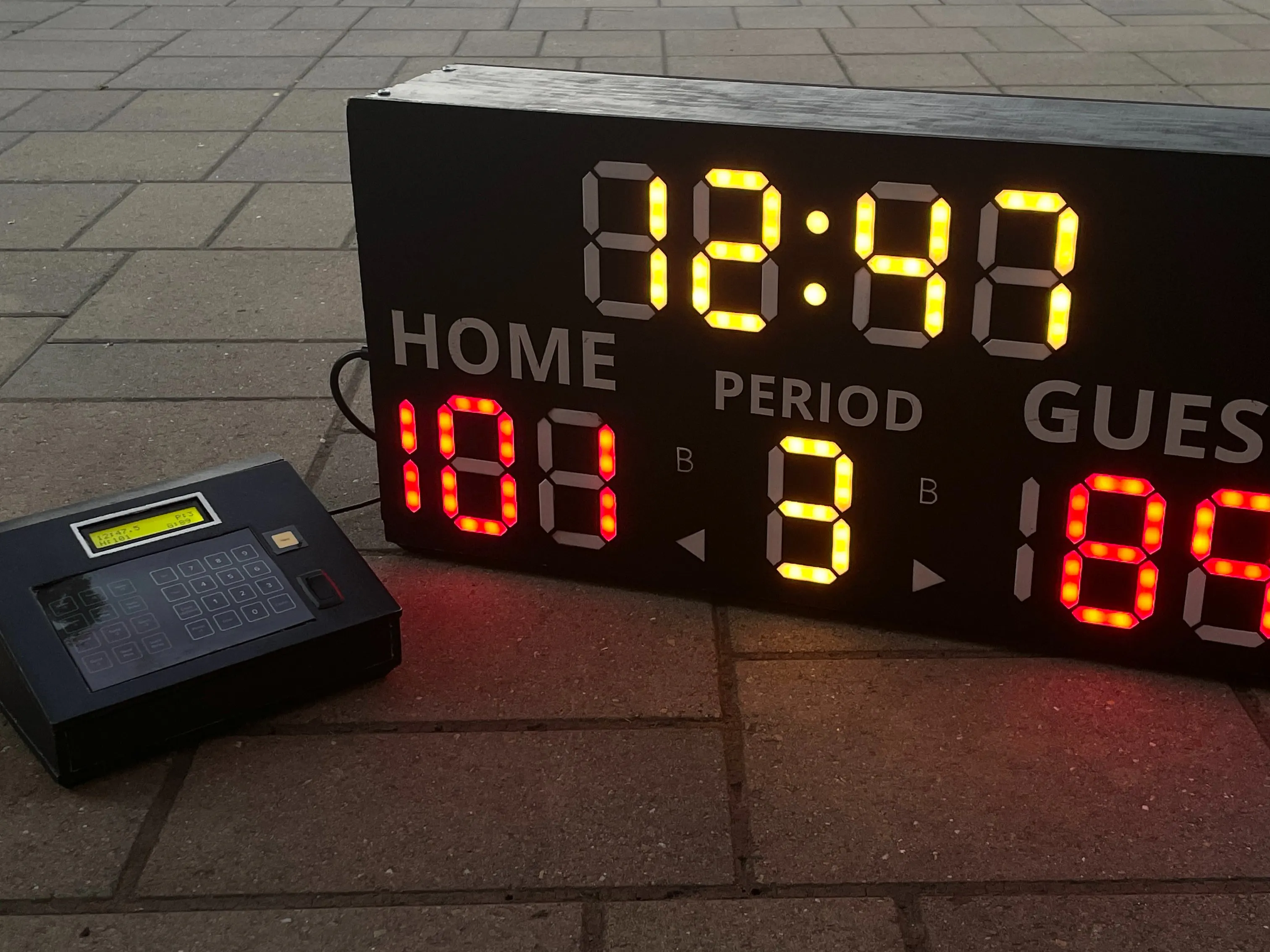## Things used in this project

### Hardware componentsArduino UNO
×1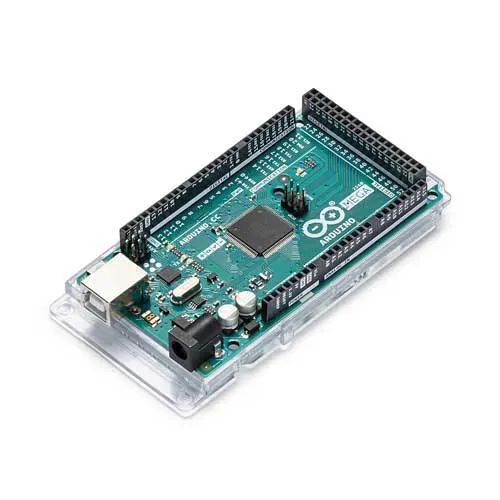Arduino Mega 2560
×1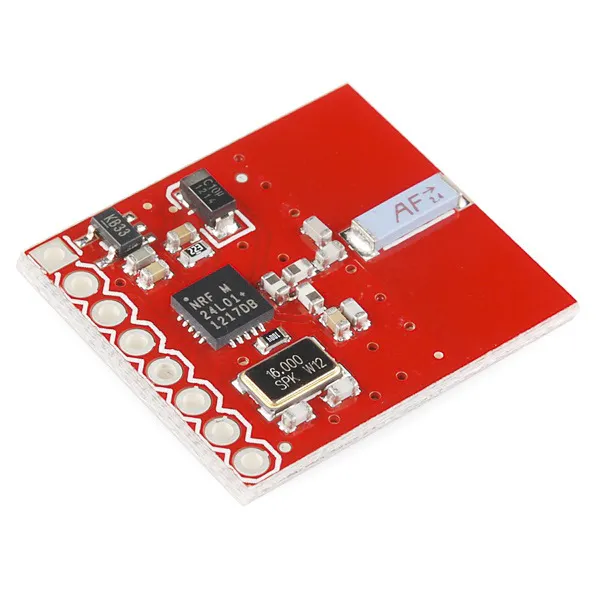SparkFun Transceiver Breakout - nRF24L01+
×2
 WS2812B LED Strip (5 meters, 60 LED/m)
×1
 24" x 12" MDF (or wood equivalent)
×2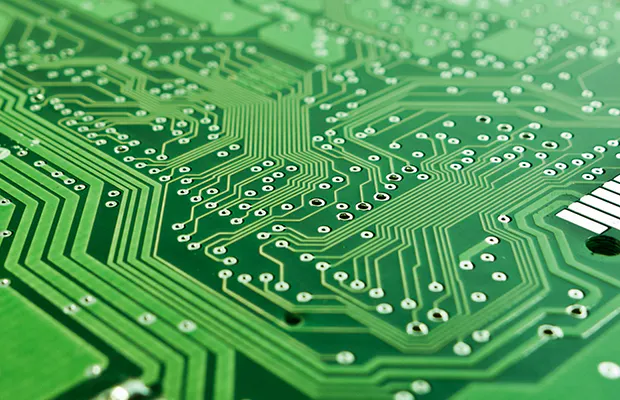Custom PCB
×2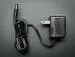9V 1A Switching Wall Power Supply
×2
 5v 20A Power Supply
×1
 Rocker Switch
×2
 16 x 2 Character LCD
×1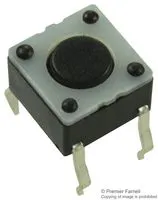Tactile Switch, Top Actuated
×29
 White Vinyl
×1
 Federal Signal Vibratone 350 Horn
×1

### Software apps and online servicesArduino IDE

### Hand tools and fabrication machinesSoldering iron (generic)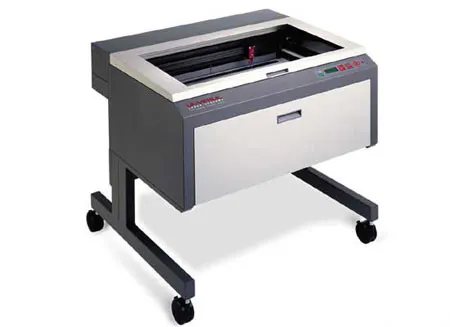Laser cutter (generic)Hot glue gun (generic)3D Printer (generic)

## Schematics

### Scoreboard Schematic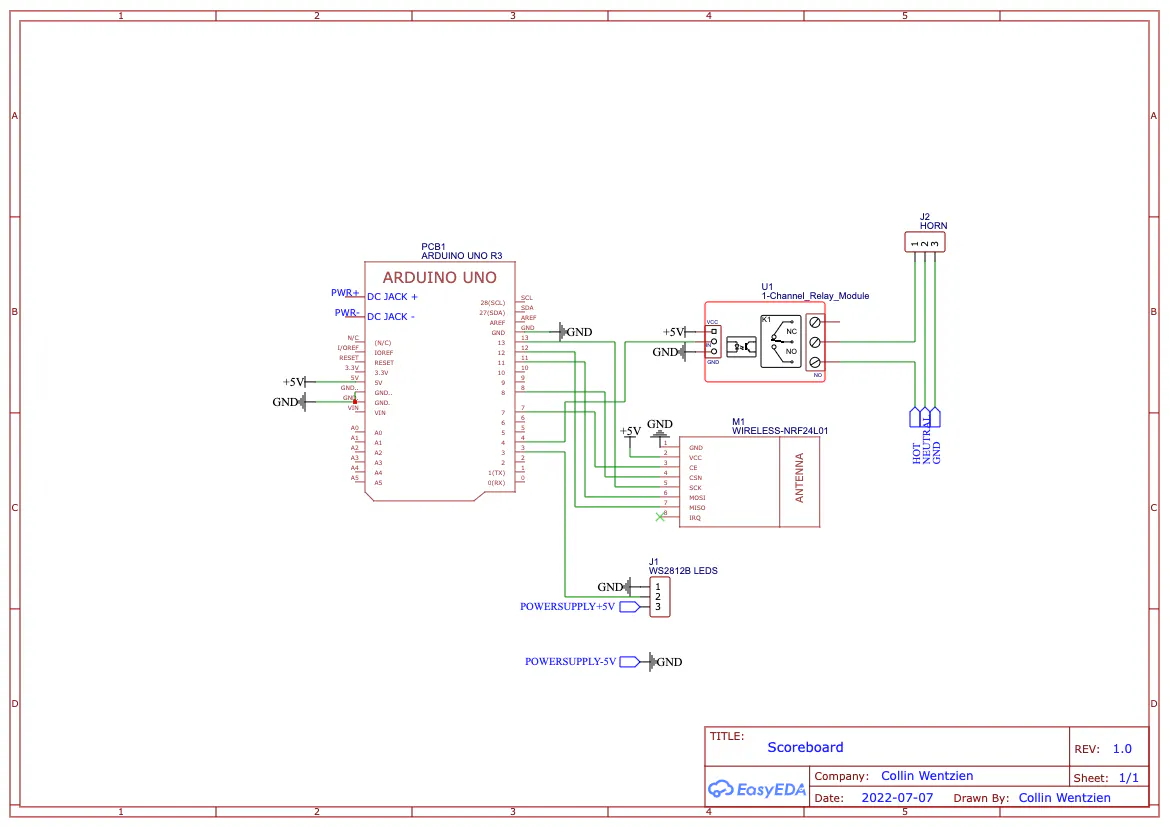### Controller Schematic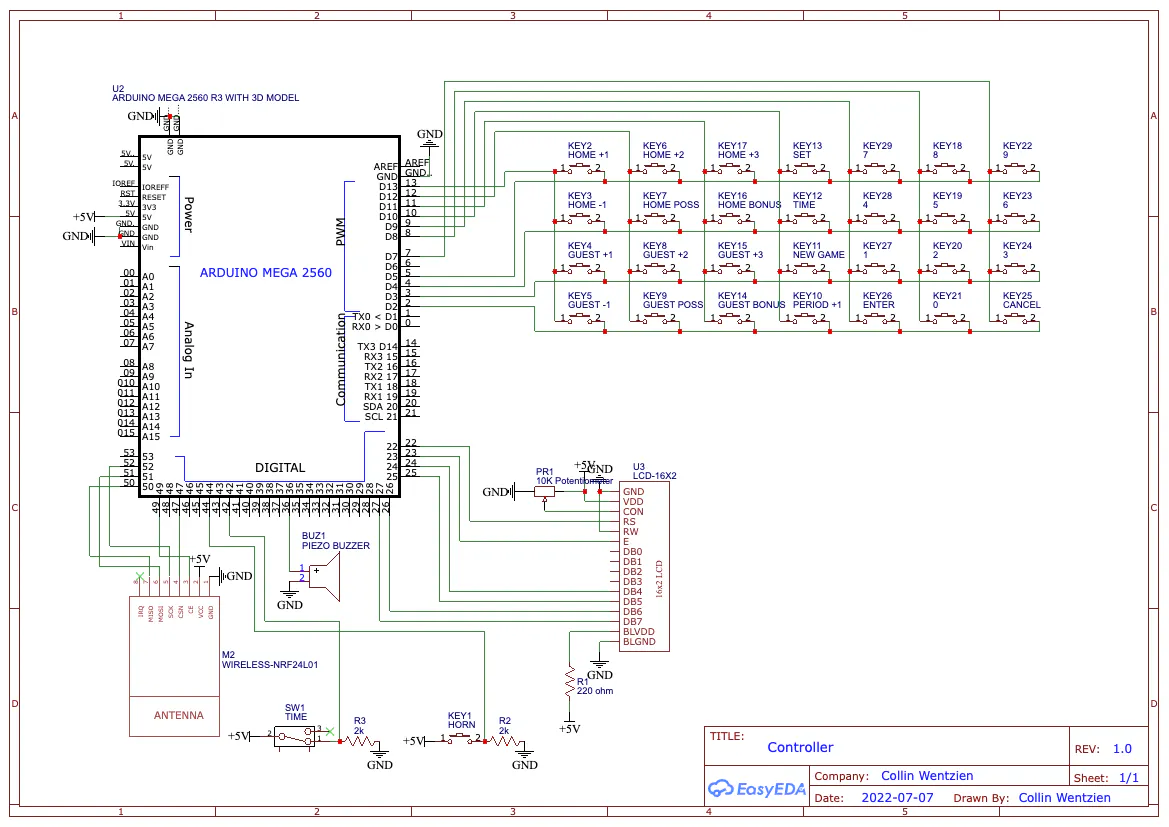## Code

### Scoreboard Code

Arduino
Code for scoreboard. Receives all instructions from controller and displays on scoreboard
```/*
*
*  Timetronics Controller Rev. 0.1
*  Running Timetroinics OS v1.2 (latest build 3/28/2022)
*  Designed and built by Collin Wentzien
*
*  Compatible with 24" x 12" scoreboards with 2 LED segments
*
*/

// -------------------- Initialization -------------------- //
// ---------- LEDs ----------//
#include <FastLED.h>
#define LED_PIN     3
#define NUM_LEDS    140
#include <SPI.h>

CRGB leds[NUM_LEDS];

// ---------- Radio ----------//
#include <nRF24L01.h>
#include <RF24.h>

RF24 radio(7, 8); // CE, CSN
const byte address = "00001";

// ---------- Other ---------- //
const int buzzerPin = 4;
boolean horn = false;
int times = 0;

int charLayout = {
{0, 0, 1, 1, 1, 1, 1, 1, 1, 1, 1, 1, 1, 1}, //0
{0, 0, 1, 1, 0, 0, 0, 0, 0, 0, 0, 0, 1, 1}, //1
{1, 1, 0, 0, 1, 1, 1, 1, 0, 0, 1, 1, 1, 1}, //2
{1, 1, 1, 1, 1, 1, 0, 0, 0, 0, 1, 1, 1, 1}, //3
{1, 1, 1, 1, 0, 0, 0, 0, 1, 1, 0, 0, 1, 1}, //4
{1, 1, 1, 1, 1, 1, 0, 0, 1, 1, 1, 1, 0, 0}, //5
{1, 1, 1, 1, 1, 1, 1, 1, 1, 1, 1, 1, 0, 0}, //6
{0, 0, 1, 1, 0, 0, 0, 0, 0, 0, 1, 1, 1, 1}, //7
{1, 1, 1, 1, 1, 1, 1, 1, 1, 1, 1, 1, 1, 1}, //8
{1, 1, 1, 1, 0, 0, 0, 0, 1, 1, 1, 1, 1, 1}, //9
{0, 0, 0, 0, 0, 0, 0, 0, 0, 0, 0, 0, 0, 0}  //CLR
} ;

//does not include the 1 in third score digit and colon and bonus / poss
int offset[] = {0, 14, 30, 44, 62, 76, 92, 112, 126};

// -------------------- Setup -------------------- //
void setup() {
FastLED.addLeds<WS2812, LED_PIN, GRB>(leds, NUM_LEDS);

Serial.begin(9600);

pinMode(buzzerPin, OUTPUT);

clearScreen();

//Draw colon
leds = CRGB(255, 120, 0);
leds = CRGB(255, 120, 0);
}

// -------------------- Draw Digit -------------------- //
void drawChar(int number, int offsetY, String color) {
for (int i = 0; i <= 13; i++) {
if(charLayout[number][i] == 1) {//chose num
if(color == "red") {
leds[i + offset[offsetY]] = CRGB (220, 0, 0);
}else if(color == "yellow") {
leds[i + offset[offsetY]] = CRGB (255, 120, 0);
}
}else {
leds[i + offset[offsetY]] = CRGB (0, 0, 0);
}
}
}

// -------------------- Draw Other Character -------------------- //
void drawOther(String item) {
if(item == "hOne") {
for(int i = 58; i <= 61; i++) {
leds[i] = CRGB(220, 0, 0);
}

}else if(item == "hClear") {
for(int i = 58; i <= 61; i++) {
leds[i] = CRGB(0, 0, 0);
}

}else if(item == "gOne") {
for(int i = 108; i <= 111; i++) {
leds[i] = CRGB(220, 0, 0);
}

}else if(item == "gClear") {
for(int i = 108; i <= 111; i++) {
leds[i] = CRGB(0, 0, 0);
}
}

}

// -------------------- Clear Digit -------------------- //
void clearScreen() {
for (int i = 0; i <= 139; i++) {
leds[i] = CRGB(0, 0, 0);
}
FastLED.show();
}

// -------------------- Loop -------------------- //
void loop() {
if(times >= 10) {
horn = false;
times = 0;
}else{
times++;
}

Serial.println(times);

char clk = "";

if(String(clk) == "t") {

//Milliseconds (08.6)
if((clk - 0) - '0' == 0 && (clk - 0) - '0' == 0) {
leds = CRGB(0, 0, 0);
leds = CRGB(255, 120, 0);

drawChar(10, 3, "");

drawChar((clk - 0) - '0', 0, "yellow");
drawChar((clk - 0) - '0', 1, "yellow");

drawChar((clk - 0) - '0', 2, "yellow");

FastLED.show();

//Regular (19:50)
}else{
leds = CRGB(255, 120, 0);
leds = CRGB(255, 120, 0);

if((clk - 0) - '0' != 0) {
drawChar((clk - 0) - '0', 0, "yellow");
}else{
drawChar(10, 0, "");
}
drawChar((clk - 0) - '0', 1, "yellow");
drawChar((clk - 0) - '0', 2, "yellow");
drawChar((clk - 0) - '0', 3, "yellow");

FastLED.show();

}
}

if(String(clk) == "h") {
if((clk - 0) - '0' != 0) {
drawOther("hOne");
}else{
drawOther("hClear");
}

if(((clk - 0) - '0' == 0) && ((clk - 0) - '0' == 0)) {
drawChar(10, 4, "");

}else{
drawChar((clk - 0) - '0', 4, "red");
}

drawChar((clk - 0) - '0', 5, "red");

FastLED.show();
}

if(String(clk) == "g") {
if((clk - 0) - '0' != 0) {
drawOther("gOne");
}else{
drawOther("gClear");
}

if(((clk - 0) - '0' == 0) && ((clk - 0) - '0' == 0)) {
drawChar(10, 7, "");

}else{
drawChar((clk - 0) - '0', 7, "red");
}

drawChar((clk - 0) - '0', 8, "red");

FastLED.show();
}

if(String(clk) == "p") {
drawChar((clk - 0) - '0', 6, "yellow");

FastLED.show();
}

if(String(clk) == "a") { //home bonus
if((clk - 0) - '0' == 1) {
leds = CRGB(255, 120, 0);
}else{
leds = CRGB(0, 0, 0);
}

FastLED.show();
}

if(String(clk) == "b") { //guest bonus
if((clk - 0) - '0' == 1) {
leds = CRGB(255, 120, 0);
}else{
leds = CRGB(0, 0, 0);
}

FastLED.show();
}

if(String(clk) == "c" ) { //home poss
if((clk - 0) - '0' == 1) {
leds = CRGB(220, 0, 0);
}else{
leds = CRGB(0, 0, 0);
}

FastLED.show();
}

if(String(clk) == "d") { //guest poss
if((clk - 0) - '0' == 1) {
leds = CRGB(220, 0, 0);
}else{
leds = CRGB(0, 0, 0);
}

FastLED.show();
}

if(String(clk) == "o") {
horn = true;
times = 0;
}else{
horn = false;
}

}

Serial.println(horn);

if(horn == true) {
digitalWrite(buzzerPin, HIGH);
}else{
digitalWrite(buzzerPin, LOW);
}
}
```

### Controller Code

Arduino
Code for controller. (Mostly) bug free.
```/*
*
*  Timetronics Controller Rev. 0.1
*  Running Timetroinics OS v1.2 (latest build 12/3/2021)
*  Designed and built by Collin Wentzien
*
*  Compatible with 24" x 12" scoreboards with 2 LED segments
*
*/

// -------------------- Initialization -------------------- //
// ---------- LCD ----------//
#include <LiquidCrystal.h>
LiquidCrystal lcd(22, 23, 24, 25, 26, 27);

byte hornChar = {
B00000,
B11111,
B10001,
B10101,
B10001,
B11111,
B00000,
B00000
};

// ---------- Radio ---------- //
#include <RF24.h>
#include <nRF24L01.h>

RF24 radio(49, 47); // CE, CSN
const byte address = "00001";

// ---------- Keypad ---------- //
#include <SPI.h>

const byte ROWS = 4;
const byte COLS = 7;

char keys [ROWS] [COLS] = {
{'a', 'b', 'c',     's', '7', '8', '9'},
{'d', 'e', 'f',     't', '4', '5', '6'},
{'g', 'h', 'i',     'u', '1', '2', '3'},
{'j', 'k', 'l',     'p', 'y', '0', 'n'}
};

byte rowPins[ROWS] = {5, 4, 3, 2};
byte colPins[COLS] = {13, 12, 11, 10, 9, 8, 7};

// ---------- I/O ---------- //
const int timeToggle = 42;
const int buzzer = 40;
const int hornBtn = 44;

int buttonState = 0;
int hornState = 0;

// ---------- Timing ---------- //
String M1, M2, S1, S2, s;
int m1,m2,s1,s2,S;

unsigned long currentTime;
unsigned long previousTime;

int numLength = 0;
String num1="";
String num2="";
String num3="";
String num4="";
String num5="";
String num6="";
String num7="";

unsigned long currentHorn;
unsigned long previousHorn;

unsigned long currentScreen;
unsigned long previousScreen;
boolean isHome;
boolean first = true;

// ---------- Score ---------- //
String gScore; // to figure out where G: sits on hs
int oldHScore, oldGScore, oldPeriod;
int homeScore = 0;
int guestScore = 0;

// ---------- Poss and Bonus ---------- //
boolean homePoss = false;
boolean homeBonus = false;
boolean guestPoss = false;
boolean guestBonus = false;

// ---------- Period ---------- //
int period = 1;

// ---------- Horn ---------- //
int buzzTime;
int hornTime;

boolean horn = false;
boolean hhorn = false;

// ---------- Other ---------- //
#include <EEPROM.h>
boolean autohorn = true;

boolean timer, hscore, gscore, periods, ct;

boolean selected = false;
boolean pressed = false;
boolean updated = false;
boolean used = false;
boolean on = false;
boolean down = true;
boolean zeroed = false;

// -------------------- Setup -------------------- //
void setup() {
Serial.begin(9600);

if(EEPROM.read(0) != 0 || EEPROM.read(0) != 1)
EEPROM.write(0, 1);
autohorn = true;
else
autohorn = false;

lcd.begin(16,2);
lcd.createChar(0, hornChar);
lcd.clear();

pinMode(timeToggle, INPUT);
pinMode(hornBtn, INPUT);
pinMode(buzzer, OUTPUT);

lcd.setCursor(2,0);
lcd.print("Timetronics");
lcd.setCursor(2,1);
lcd.print("Scoreboards");

digitalWrite(buzzer, HIGH);
delay(400);
digitalWrite(buzzer, LOW);

delay(700);
lcd.clear();
delay(100);

M1="1";
M2="5";
S1="0";
S2="0";
s="0";

sendData("time");
sendData("hScore");
sendData("gScore");
sendData("period");

updated=true;
hhorn = false;
homeScreen();
}

// -------------------- Home Screen ------------------- //
void homeScreen() {
isHome = true;
pressed=false;
gScore = guestScore;
int numLength = gScore.length();
lcd.setCursor(0,0);
if(M1 != "0") {
lcd.print(M1);
lcd.print(M2);
lcd.print(":");
lcd.print(S1);
lcd.print(S2);
lcd.print(".");
lcd.print(s);

if(horn == true) {
lcd.setCursor(7, 0);
lcd.write(byte(0));

}else{
lcd.setCursor(7, 0);
lcd.print(" ");
}

}else{
lcd.print(M2);
lcd.print(":");
lcd.print(S1);
lcd.print(S2);
lcd.print(".");
lcd.print(s);

if(horn == true) {
lcd.setCursor(6, 0);
lcd.write(byte(0));

}else{
lcd.setCursor(6, 0);
lcd.write(" ");
}
}

lcd.setCursor(13,0);
lcd.print("P:");
lcd.setCursor(15,0);
lcd.print(period);

lcd.setCursor(0,1);
lcd.print("H:");
lcd.setCursor(2,1);
lcd.print(homeScore);
lcd.setCursor(11,1);
lcd.print("G:");
lcd.setCursor(13,1);
lcd.print(guestScore);

lcd.setCursor(7,1);
if(homePoss)
lcd.print("<");
else if(guestPoss)
lcd.print(">");

if(homeBonus) {
lcd.setCursor(6,1);
lcd.print("B");
}
if(guestBonus) {
lcd.setCursor(8,1);
lcd.print("B");
}
}

// -------------------- Loop -------------------- //
void loop() {
char key = myKeypad.getKey();

//mini buzzer for controller
if(key != NO_KEY && key != 'o' && key != 'g') {
for(int i = 0; i < 1000; i++) {
digitalWrite(buzzer,HIGH);
}
digitalWrite(buzzer,LOW);
}

if(isHome == false) {
updated = false;

currentScreen = millis();
if(currentScreen - previousScreen >= 2000) {
Serial.println(currentScreen - previousScreen);
previousScreen = millis();
lcd.clear();
updated = true;
homeScreen();

}
}

//TIME
m1=M1.toInt();
m2=M2.toInt();
s1=S1.toInt();
s2=S2.toInt();
S=s.toInt();

if(buttonState==LOW) {
if(m1==0 && m2==0 && s1==0 && s2==0 && S==0) { //AUTOHORN
if(zeroed == false) {
previousHorn=millis();
sendData("time");
zeroed = true;
}

currentHorn=millis();
if(currentHorn-previousHorn<=2500 && autohorn == true) {
sendData("horn");
horn = true;

}else{
if(zeroed == false) {
previousHorn=millis();
horn = false;
}
}

}else{
currentTime=millis();
if(currentTime-previousTime>=94) {
S=S-1;
previousTime=millis();
}

if(S<0) {
S=9;
s2=s2-1;
s=String(S);
S2=String(s2);
}if(s2<0) {
s2=9;
s1=s1-1;
S2=String(s2);
S1=String(s1);
}if(s1<0) {
s1=5;
m2=m2-1;
S1=String(s1);
M2=String(m2);
}if(m2<0) {
m2=9;
m1=m1-1;
M1=String(m1);
M2=String(m2);
}if(m1<0) {
m1=0;
M1=String(m1);
}

sendData("time"); //more accurate, but uses more power
}
}

M1=String(m1);
M2=String(m2);
S1=String(s1);
S2=String(s2);
s=String(S);

//HOME
//  if(key=='t' && pressed==false) {
//    updated = true;
//    lcd.clear();
//    homeScreen();
//  }

if(updated==true) {
homeScreen();
}

//SET

if(key=='s'){
updated = false;
pressed = true;
selected = true;
lcd.clear();
lcd.setCursor(0,0);
lcd.print("Set...");
}

//time
if(key=='t' && pressed==true && selected == true){
if(buttonState == LOW) {
selected = false;
lcd.clear();
lcd.setCursor(0,0);
lcd.print("Stop time before");
lcd.setCursor(0,1);
lcd.print("setting time");

}else {
zeroed = false;
selected = false;
num4="";
num7="";

M1="";
M2="";
S1="";
S2="";
s="";
timer=true;
lcd.clear();
lcd.setCursor(0,0);
lcd.print("Set Time:");
lcd.setCursor(0,1);
lcd.print("MM:SS.s");
}

}else if(key=='a' && pressed==true && selected == true) {
selected = false;
num1 = "";
hscore=true;
lcd.clear();
lcd.setCursor(0,0);
lcd.print("Set Home Score:");
lcd.setCursor(0,1);
lcd.print(homeScore);

sendData("hScore");

}else if(key=='g' && pressed==true && selected == true) {
selected = false;
num2 = "";
gscore=true;
lcd.clear();
lcd.setCursor(0,0);
lcd.print("Set Guest Score:");
lcd.setCursor(0,1);
lcd.print(guestScore);

sendData("gScore");

}else if(key=='p' && pressed==true && selected == true) {
selected = false;
num3 = "";
periods=true;
lcd.clear();
lcd.setCursor(0,0);
lcd.print("Set Period:");
lcd.setCursor(0,1);
lcd.print(period);

sendData("period");
}

if(key!=NO_KEY && key=='0' || key=='1' || key=='2' || key=='3' || key=='4' || key=='5' || key=='6' || key=='7' || key=='8' || key=='9' && pressed==true) {
updated = false;
if(hscore==true) {
numLength = num1.length();
if(numLength<3) {
num1 = num1 + key;
lcd.clear();
lcd.setCursor(0,0);
lcd.print("Set Home Score:");
lcd.setCursor(0,1);
lcd.print(num1);
}
}
if(gscore==true) {
numLength = num2.length();
if(numLength<3) {
num2 = num2 + key;
lcd.clear();
lcd.setCursor(0,0);
lcd.print("Set Guest Score:");
lcd.setCursor(0,1);
lcd.print(num2);
}
}
if(periods==true) {
num3 = key;
lcd.clear();
lcd.setCursor(0,0);
lcd.print("Set Period:");
lcd.setCursor(0,1);
lcd.print(num3);
}
if(timer==true) {
num7=num7+key;
numLength = num7.length();
if(numLength==1) {
s=key;
lcd.setCursor(6,1);
lcd.print(s);

}else if(numLength==2) {
S2=s;
s=key;
lcd.setCursor(4,1);
lcd.print(S2);
lcd.print(".");
lcd.print(s);

}else if(numLength==3) {
S1=S2;
S2=s;
s=key;
lcd.setCursor(3,1);
lcd.print(S1);
lcd.print(S2);
lcd.print(".");
lcd.print(s);

}else if(numLength==4) {
M2=S1;
S1=S2;
S2=s;
s=key;
lcd.setCursor(1,1);
lcd.print(M2);
lcd.print(":");
lcd.print(S1);
lcd.print(S2);
lcd.print(".");
lcd.print(s);

}else if(numLength==5) {
M1=M2;
M2=S1;
S1=S2;
S2=s;
s=key;
lcd.setCursor(0,1);
lcd.print(M1);
lcd.print(M2);
lcd.print(":");
lcd.print(S1);
lcd.print(S2);
lcd.print(".");
lcd.print(s);
}
}
}

if(key!=NO_KEY && key=='y' && pressed==true) {
if(S1=="6" || S1=="7" || S1=="8" || S1=="9") {
lcd.clear();
lcd.setCursor(0,0);
lcd.print("Invalid time");
M1="2";
M2="0";
S1="0";
S2="0";
s="0";

sendData("time");

pressed=false;
periods=false;
hscore=false;
gscore=false;
timer=false;
updated=true;
ct=false;
used=false;
hhorn = true;
lcd.clear();
homeScreen();

hhorn = true;

}else{
used=false;
if(periods==true) {
period = num3.toInt();
}else if(hscore==true) {
homeScore = num1.toInt();
}else if(gscore==true) {
guestScore = num2.toInt();
}
pressed=false;
periods=false;
hscore=false;
gscore=false;
timer=false;
ct=false;
lcd.clear();
homeScreen();
updated=true;

hhorn = true;
}

sendData("time");
sendData("hScore");
sendData("gScore");
sendData("period");

}

if(key!=NO_KEY && key=='n'&& pressed==true) {

sendData("time");
sendData("hScore");
sendData("gScore");
sendData("period");

pressed=false;
periods=false;
hscore=false;
gscore=false;
timer=false;
updated=true;
ct=false;
used=false;
hhorn = true;
lcd.clear();
homeScreen();
}

//SCORE
//Serial.println(buttonState); //for debugging purposes

//home
if(key == 'a' && pressed==false && homeScore < 199) {
updated = false;
homeScore += 1;
lcd.clear();
lcd.setCursor(0,0);
lcd.print("Home Score +1");
lcd.setCursor(0,1);
lcd.print(homeScore);

sendData("hScore");

previousScreen = millis();
isHome = false;

}else if (key == 'b' && pressed==false && homeScore < 198) {
updated = false;
homeScore += 2;
lcd.clear();
lcd.setCursor(0,0);
lcd.print("Home Score +2");
lcd.setCursor(0,1);
lcd.print(homeScore);

sendData("hScore");

previousScreen = millis();
isHome = false;

}else if (key == 'c' && pressed==false && homeScore < 197) {
updated = false;
homeScore += 3;
lcd.clear();
lcd.setCursor(0,0);
lcd.print("Home Score +3");
lcd.setCursor(0,1);
lcd.print(homeScore);

sendData("hScore");

previousScreen = millis();
isHome = false;

}else if (key == 'd' && pressed==false && homeScore!=0) {
updated = false;
homeScore -= 1;
lcd.clear();
lcd.setCursor(0,0);
lcd.print("Home Score -1");
lcd.setCursor(0,1);
lcd.print(homeScore);

sendData("hScore");

previousScreen = millis();
isHome = false;

//guest
}else if (key == 'g' && pressed==false && guestScore < 199) {
updated = false;
guestScore += 1;
lcd.clear();
lcd.setCursor(0,0);
lcd.print("Guest Score +1");
lcd.setCursor(0,1);
lcd.print(guestScore);

sendData("gScore");

previousScreen = millis();
isHome = false;

}else if (key == 'h' && pressed==false && guestScore < 198) {
updated = false;
guestScore += 2;
lcd.clear();
lcd.setCursor(0,0);
lcd.print("Guest Score +2");
lcd.setCursor(0,1);
lcd.print(guestScore);

sendData("gScore");

previousScreen = millis();
isHome = false;

}else if (key == 'i' && pressed==false && guestScore < 197) {
updated = false;
guestScore += 3;
lcd.clear();
lcd.setCursor(0,0);
lcd.print("Guest Score +3");
lcd.setCursor(0,1);
lcd.print(guestScore);

sendData("gScore");

previousScreen = millis();
isHome = false;

}else if (key == 'j' && pressed==false && guestScore!=0) {
updated = false;
guestScore -= 1;
lcd.clear();
lcd.setCursor(0,0);
lcd.print("Guest Score -1");
lcd.setCursor(0,1);
lcd.print(guestScore);

sendData("gScore");

previousScreen = millis();
isHome = false;

}

//POSS AND BONUS
if(key == 'e' && pressed==false) {
updated = false;
homePoss = !homePoss;
if(guestPoss)
guestPoss = !guestPoss;
lcd.clear();
lcd.setCursor(0,0);
lcd.print("Home Possession");
lcd.setCursor(0,1);
lcd.print(homePoss ? "On" : "Off");

sendData("c");
sendData("d");

previousScreen = millis();
isHome = false;

} else if(key == 'k' && pressed==false) {
updated = false;
guestPoss = !guestPoss;
if(homePoss)
homePoss = !homePoss;
lcd.clear();
lcd.setCursor(0,0);
lcd.print("Guest Possession");
lcd.setCursor(0,1);
lcd.print(guestPoss ? "On" : "Off");

sendData("c");
sendData("d");

previousScreen = millis();
isHome = false;

}

if(key == 'f' && pressed==false) {
updated = false;
homeBonus = !homeBonus;
lcd.clear();
lcd.setCursor(0,0);
lcd.print("Home Bonus");
lcd.setCursor(0,1);
lcd.print(homeBonus ? "On" : "Off");

sendData("a");

previousScreen = millis();
isHome = false;

} else if(key == 'l' && pressed==false) {
updated = false;
guestBonus = !guestBonus;
lcd.clear();
lcd.setCursor(0,0);
lcd.print("Guest Bonus");
lcd.setCursor(0,1);
lcd.print(guestBonus ? "On" : "Off");

sendData("b");

previousScreen = millis();
isHome = false;
}

//PERIOD
if(key=='p' && pressed==false) {
updated = false;
period += 1;
if(period<=9) {
}else{
period = 0;
}
lcd.clear();
lcd.setCursor(0,0);
lcd.print("Period +1");
lcd.setCursor(0,1);
lcd.print(period);

sendData("period");

previousScreen = millis();
isHome = false;

}

//  if(key == 'u' && pressed == false) {
//    digitalWrite(buzzer, HIGH);
//    delay(400);
//    digitalWrite(buzzer, LOW);
//
//    zeroed = false;
//    lcd.clear();
//    delay(400);
//
//    M1="1";
//    M2="5";
//    S1="0";
//    S2="0";
//    s="0";
//
//    homeScore = 0;
//    guestScore = 0;
//    period = 1;
//
//    sendData("time");
//    sendData("hScore");
//    sendData("gScore");
//    sendData("period");
//
//    homeScreen();
//  }

if(key == 'u' && pressed == false) {
updated = false;

if(autohorn) {
autohorn = false;
EEPROM.write(0, 0);
} else {
autohorn = true;
EEPROM.write(0, 1);
}

lcd.clear();
lcd.setCursor(0,0);
lcd.print("Auto Horn");
lcd.setCursor(0,1);
lcd.print(autohorn ? "On" : "Off");

previousScreen = millis();
isHome = false;
}

//HORN
if(hornState == HIGH) {
horn = true;
sendData("horn");

}else{
horn = false;
}
}

// -------------------- Send Data -------------------- //
void sendData(String item) {
if(item == "time") {
String all = "t" + M1 + M2 + ":" + S1 + S2 + "." + s;
char clk;
all.toCharArray(clk, 32);

}else if(item == "hScore") {
String hTot;

if(homeScore < 10) {
hTot = "h00" + String(homeScore);
}else if(homeScore < 100) {
hTot = "h0" + String(homeScore);
}else{
hTot = "h" + String(homeScore);
}

char hSc;
hTot.toCharArray(hSc, 32);

}else if(item == "gScore") {
String gTot;

if(guestScore < 10) {
gTot = "g00" + String(guestScore);
}else if(guestScore < 100) {
gTot = "g0" + String(guestScore);
}else{
gTot = "g" + String(guestScore);
}

char gSc;
gTot.toCharArray(gSc, 32);

}else if(item == "period") {
String pTot = "p" + String(period);

char pSc;
pTot.toCharArray(pSc, 32);

}else if(item == "horn") {
char hrn = "o";

}else if(item == "a") {
String total = "a" + String(homeBonus);
char snd;
total.toCharArray(snd, 32);

}else if(item == "b") {
String total = "b" + String(guestBonus);
char snd;
total.toCharArray(snd, 32);

}else if(item == "c") {
String total = "c" + String(homePoss);
char snd;
total.toCharArray(snd, 32);

}else if(item == "d") {
String total = "d" + String(guestPoss);
char snd;
total.toCharArray(snd, 32);